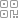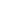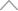• 找新知
• 找服务/产品
• 找课程
• 找LIVE
• 找活动
• 找达人海量新知2 2 2 6 1 1 8

# 通过do_execve源码分析程序的执行（上）（基于linux0.11）

execve函数是操作系统非常重要的一个函数，他使得程序变成进程成为可能。下面我们通过do_execve的实现，了解一下程序变成进程的过程。首先do_execve是一个系统调用。之前分析过系统调用的过程。这里就不详细说了。直接从sys_execve函数开始。

_sys_execve:

lea EIP(%esp),%eax

pushl %eax

call _do_execve

retint do_execve(unsigned long * eip,long tmp,char * filename,

char ** argv, char ** envp)

{

struct m_inode * inode;

struct exec ex;

unsigned long page[MAX_ARG_PAGES];

int i,argc,envc;

int e_uid, e_gid;

int retval;

int sh_bang = 0;

unsigned long p=PAGE_SIZE*MAX_ARG_PAGES-4;

// eip指向系统调用前的eip，eip则指向cs，判断一下这时候的cs是不是用户的cs

if ((0xffff & eip) != 0x000f)

panic("execve called from supervisor mode");

for (i=0 ; i/* clear page-table */

page[i]=0;

// 通过文件名找到可执行文件

if (!(inode=namei(filename)))       /* get executables inode */

return -ENOENT;

// 计算环境变量和参数个数

argc = count(argv);

envc = count(envp);

restart_interp:

if (!S_ISREG(inode->i_mode)) {  /* must be regular file */

retval = -EACCES;

goto exec_error2;

}

i = inode->i_mode;

// 设置了uid则执行的时候uid是设置的uid，否则是用户的有效id

e_uid = (i & S_ISUID) ? inode->i_uid : current->euid;

e_gid = (i & S_ISGID) ? inode->i_gid : current->egid;

// 相等说明该文件是该用户创建的，则判断user位的权限

if (current->euid == inode->i_uid)

i >>= 6;

// 同上，判断组权限

else if (current->egid == inode->i_gid)

i >>= 3;

/*

else 判断 other的权限

*/

if (!(i & 1) &&

!((inode->i_mode & 0111) && suser())) {

retval = -ENOEXEC;

goto exec_error2;

}

// 读第一块数据进来

retval = -EACCES;

goto exec_error2;

}

// 前面是执行文件的头，包括一些元数据

// 是脚脚本文件，不是编译后的文件,sh_bang控制只会进入一次

if ((bh->b_data == '#') && (bh->b_data == '!') && (!sh_bang)) {

/*

* This div does the #! interpretation.

* Sorta complicated, but hopefully it will work.  -TYT

*/

char buf, *cp, *interp, *i_name, *i_arg;

unsigned long old_fs;

// 把#!之外的字符复制到buf

strncpy(buf, bh->b_data+21022);

brelse(bh);

iput(inode);

buf = '';

// 找出buf里第一次出现换行字符的地址，没有则返回NULL

if (cp = strchr(buf, ' ')) {

// 更新换行字符为，表示字符串结束

*cp = '';

// cp指向文件的第一个字符

for (cp = buf; (*cp == ' ') || (*cp == 't'); cp++);

}

if (!cp || *cp == '') {

retval = -ENOEXEC; /* No interpreter name found */

goto exec_error1;

}

// 开始找出解释器名字

interp = i_name = cp;

i_arg = 0;

// interp指向解释器路径的第一个字符，iname指向解释器名称

for ( ; *cp && (*cp != ' ') && (*cp != 't'); cp++) {

if (*cp == '/')

i_name = cp+1;

}

// 遇到空格或制表符结束的，则修改他的值为

if (*cp) {

*cp++ = '';

// i_arg指向解释器名称的字符，即参数列表

i_arg = cp;

}

/*

* OK, we've parsed out the interpreter name and

* (optional) argument.

*/

// sh_bang初始值是0，加一，用作下面代码判断的标记，见下一个sh_bang变量

if (sh_bang++ == 0) {

p = copy_strings(envc, envp, page, p, 0);

p = copy_strings(--argc, argv+1, page, p, 0);

}

/*

* Splice in (1) the interpreter's name for argv

*           (2) (optional) argument to interpreter

*           (3) filename of shell script

*

* This is done in reverse order, because of how the

* user environment and arguments are stored.

*/

// 脚本的名字

p = copy_strings(1, &filename, page, p, 1);

argc++;

// 解释器的参数列表

if (i_arg) {

p = copy_strings(1, &i_arg, page, p, 2);

argc++;

}

// 解释器名字

p = copy_strings(1, &i_name, page, p, 2);

argc++;

if (!p) {

retval = -ENOMEM;

goto exec_error1;

}

/*

* OK, now restart the process with the interpreter's inode.

*/

old_fs = get_fs();

set_fs(get_ds());

if (!(inode=namei(interp))) { /* get executables inode */

set_fs(old_fs);

retval = -ENOENT;

goto exec_error1;

}

set_fs(old_fs);

// 复制完，加载解释器的可执行文件

goto restart_interp;

}

brelse(bh);

if (N_MAGIC(ex) != ZMAGIC || ex.a_trsize || ex.a_drsize ||

ex.a_text+ex.a_data+ex.a_bss>0x3000000 ||

inode->i_size < ex.a_text+ex.a_data+ex.a_syms+N_TXTOFF(ex)) {

retval = -ENOEXEC;

goto exec_error2;

}

if (N_TXTOFF(ex) != BLOCK_SIZE) {

printk("%s: N_TXTOFF != BLOCK_SIZE. See a.out.h.", filename);

retval = -ENOEXEC;

goto exec_error2;

}

// 不是脚本文件

if (!sh_bang) {

p = copy_strings(envc,envp,page,p,0);

p = copy_strings(argc,argv,page,p,0);

// 数据太多，超过限制

if (!p) {

retval = -ENOMEM;

goto exec_error2;

}

}

/* OK, This is the point of no return */

// 替换该字段的值

if (current->executable)

iput(current->executable);

current->executable = inode;

// 清除信号处理函数

for (i=0 ; i<32 ; i++)

current->sigaction[i].sa_handler = NULL;

// 设置了close_on_exec的则关闭对应的文件

for (i=0 ; i

if ((current->close_on_exec>>i)&1)

sys_close(i);

// 清0

current->close_on_exec = 0;

// 释放代码段和数据段的页表以及物理页

free_page_tables(get_base(current->ldt),get_limit(0x0f));

free_page_tables(get_base(current->ldt),get_limit(0x17));

current->used_math = 0;

// change_ldt返回数据段的最大长度，减去MAX_ARG_PAGES*PAGE_SIZE，得到page的线性地址，加p得到p的线性地址，p是page里的偏移

p += change_ldt(ex.a_text,page)-MAX_ARG_PAGES*PAGE_SIZE;

// 复制参数和环境变量到新的地址，栈往大地址增长，p的值变大

p = (unsigned long) create_tables((char *)p,argc,envc);

// 代码、数据、bss段上面是堆指针

current->brk = ex.a_bss +

(current->end_data = ex.a_data +

(current->end_code = ex.a_text));

// p按4kb对齐成为栈指针，栈里面现在是环境变量列表和参数列表

current->start_stack = p & 0xfffff000;

// 进程的权限，setuid的时候，权限等于可执行文件拥有者的

current->euid = e_uid;

current->egid = e_gid;

i = ex.a_text+ex.a_data;

// 如果代码段和数据段的长度不是4kb的倍数（即长度的低12位有值），则把没值的部分填充0

while (i&0xfff)

put_fs_byte(0,(char *) (i++));

// 设置eip的值，返回后从这开始执行

eip = ex.a_entry;        /* eip, magic happens :-) */

// p成为栈指针即esp

eip = p;         /* stack pointer */

return 0;

exec_error2:

iput(inode);

exec_error1:

for (i=0 ; i

free_page(page[i]);

return(retval);

}

1 判断文件是否可执行和当前进程是否有权限。通过的话，加载可执行文件的第一块数据进来，只需要加载头文件就行。不需要加载具体的代码。等真正执行的时候会发送缺页中断，那时候再加载就行。

2 申请物理内存，保存环境变量和参数。具体实现在copy_string函数。

/*

* 'copy_string()' copies argument/envelope strings from user

* memory to free pages in kernel mem. These are in a format ready

* to be put directly into the top of new user memory.

*

* Modified by TYT, 11/24/91 to add the from_kmem argument, which specifies

* whether the string and the string array are from user or kernel segments:

*

* from_kmem     argv *        argv **

*    0          user space    user space

*    1          kernel space  user space

*    2          kernel space  kernel space

*

* We do this by playing games with the fs segment register.  Since it

* it is expensive to load a segment register, we try to avoid calling

* set_fs() unless we absolutely have to.

*/

static unsigned long copy_strings(int argc,char ** argv,unsigned long *page,

unsigned long p, int from_kmem)

{

char *tmp, *pag;

int len, offset = 0;

unsigned long old_fs, new_fs;

if (!p)

return 0;   /* bullet-proofing */

new_fs = get_ds();

old_fs = get_fs();

if (from_kmem==2)

set_fs(new_fs);

// 每个循环复制一个字符串

while (argc-- > 0) {

if (from_kmem == 1)

set_fs(new_fs);

// tmp指向最后一行的首地址，但是转成一级指针看起来似乎有问题

if (!(tmp = (char *)get_fs_long(((unsigned long *)argv)+argc)))

panic("argc is wrong");

if (from_kmem == 1)

set_fs(old_fs);

// 先len++表示最后的，然后每次循环加一，遇到就退出循环，不需要加一了

do {

len++;

} while (get_fs_byte(tmp++));

// 没有空间了，p从最大空间开始减

if (p-len < 0) {    /* this shouldn't happen - 128kB */

set_fs(old_fs);

return 0;

}

while (len) {

// 复制全部数据过程中，每复制一个字节p减一，tmp减一代表从字符串的后面往前面复制。

--p; --tmp; --len;

// offset是页内偏移，p是整个page数组内的偏移，p初始化的时候是4096-4，后面都是4095

if (--offset < 0) {

offset = p % PAGE_SIZE;

if (from_kmem==2)

set_fs(old_fs);

/*

从后往前复制，page的当前最后一个元素是否分配了对应的内存，

没有分配的话，给分配一页，如果数据少，可能只需要分配一页就够了

*/

if (!(pag = (char *) page[p/PAGE_SIZE]) &&

!(pag = (char *) page[p/PAGE_SIZE] =

(unsigned long *) get_free_page()))

return 0;

if (from_kmem==2)

set_fs(new_fs);

}

// 从后往前复制

*(pag + offset) = get_fs_byte(tmp);

}

}

if (from_kmem==2)

set_fs(old_fs);

return p;

}3 执行下面代码释放原来进程的页目录和页表项信息，解除到物理地址的映射。这些信息是通过fork继承下来的。

// 释放代码段和数据段的页表以及物理页

free_page_tables(get_base(current->ldt),get_limit(0x0f));

free_page_tables(get_base(current->ldt),get_limit(0x17));

4 调用change_ldt函数设置代码段、数据段的基地址和限长。数据段限长是64MB，代码段限长是根据执行文件头信息得到的。然后把page数组保存的物理地址信息映射到数据段的线性地址。

static unsigned long change_ldt(unsigned long text_size,unsigned long * page)

{

unsigned long code_limit,data_limit,code_base,data_base;

int i;

// 不够一页则占一页

code_limit = text_size+PAGE_SIZE -1;

// 4kb对齐

code_limit &= 0xFFFFF000;

// 64MB

data_limit = 0x4000000;

// 代码段和数据段的基地址是一样的，见fork.c的copy_mem

code_base = get_base(current->ldt);

data_base = code_base;

// 基地址和fork的时候是一样的，limit变了

set_base(current->ldt,code_base);

// 代码段的长度就是limit

set_limit(current->ldt,code_limit);

set_base(current->ldt,data_base);

set_limit(current->ldt,data_limit);

/* make sure fs points to the NEW data segment */

// 17是选择子，即0x10001,ldt的第三项

__asm__("pushl \$0x17 tpop %%fs"::);

// 指向数据段最后一页的末尾

data_base += data_limit;

for (i=MAX_ARG_PAGES-1 ; i>=0 ; i--) {

// 减去一页，指向数据段最后一页的首地址

data_base -= PAGE_SIZE;

/*

如果page[i]已经指向了物理地址，则建立线性地址和物理地址的映射，比如环境变量和参数

把page的最后一个元素映射到数据段的最后一页，倒数第二个元素映射到数据段倒数第二页，以此类推

*/

if (page[i])

put_page(page[i],data_base);

}

return data_limit;

}5 调用create_tables函数。分配执行环境变量和参数的数组，copy_string只是保存了环境和参数的内容。

static unsigned long * create_tables(char * p,int argc,int envc)

{

unsigned long *argv,*envp;

unsigned long * sp;

// 四个字节对齐

sp = (unsigned long *) (0xfffffffc & (unsigned long) p);

sp -= envc+1;

envp = sp;

sp -= argc+1;

argv = sp;

put_fs_long((unsigned long)envp,--sp);

put_fs_long((unsigned long)argv,--sp);

put_fs_long((unsigned long)argc,--sp);

// 复制参数到新的地址中

while (argc-->0) {

// p指向参数列表的第一个元素的地址，把这个地址存到新地址argv中

put_fs_long((unsigned long) p,argv++);

// 非空说明是参数的内容，为空，说明p++是下一个元素的地址

while (get_fs_byte(p++)) /* nothing */ ;

}

// 复制NULL给最后一个元素

put_fs_long(0,argv);

// 同上

while (envc-->0) {

put_fs_long((unsigned long) p,envp++);

while (get_fs_byte(p++)) /* nothing */ ;

}

put_fs_long(0,envp);

return sp;

}6 设置栈、堆的位置。堆位置在代码段、数据段、bss段上面。栈在p的位置（4kb对齐）。最后设置eip为执行文件头里指定的值。esp为当前的栈位置。

// 代码、数据、bss段上面是堆指针

current->brk = ex.a_bss +

(current->end_data = ex.a_data +

(current->end_code = ex.a_text));

// p按4kb对齐成为栈指针，栈里面现在是环境变量列表和参数列表

current->start_stack = p & 0xfffff000;

// 进程的权限，setuid的时候，权限等于可执行文件拥有者的

current->euid = e_uid;

current->egid = e_gid;

i = ex.a_text+ex.a_data;

// 如果代码段和数据段的长度不是4kb的倍数（即长度的低12位有值），则把没值的部分填充0

while (i&0xfff)

put_fs_byte(0,(char *) (i++));

// 设置eip的值，返回后从这开始执行

eip = ex.a_entry;        /* eip, magic happens :-) */

// p成为栈指针即esp

eip = p;         /* stack pointer */

### 新知榜

1 牛市2 逗鹅冤3 隐秘的角落4 B站5 周末随心飞6 直播带货7 中概股8 ToB9 瑞幸10 新基建•扫码下载商业新知APP~

•回到顶部